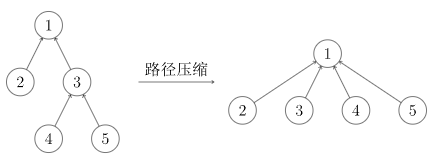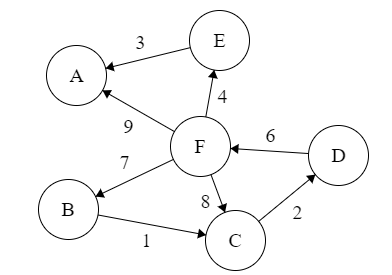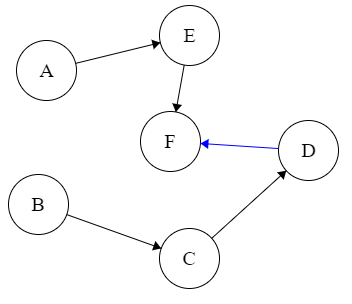## 今日目标

• 堆和堆排序
• 优先队列
• 拓扑排序和死锁检测
• 并查集
• Kruskal & Prim

## 堆

### 如何建堆

let v = [49, 38, 65, 97, 76, 13, 27, 49]
/*
49
/   \
38     65
/\       / \
 76  13  27 // 调整的起点是 97
/
49
*/


  97
/
49


）变成堆：

/*
49
/  \
38     // 然后我们处理 97 的前一个元素 65
/\     / \
49 76  13  27
/
97

49
/  \
    13 // 处理再往前一个元素
/\     / \
49 76  65  27
/
97

 // 处理再往前一个元素
/  \
38    13
/\     / \
49 76  65  27
/
97

13
/  \
38     // 如果与子节点发生了交换，那么子节点所在树要重新调整
/\     / \
49 76  65  27
/
97

13
/  \
38    27
/\     / \
49 76  65  49
/
97

*/


• 如何找到最末非叶子？就是 N/2 向下取整。
• 调整哪里？以最末非叶为根的子树调整。
• 如何调整？挑选儿子其中更小的，与自己比较然后交换！（建立小根堆，则交换使得根更小）
• 如果交换了，那么要检查交换后的子节点的树，可能需要重新调整。
• 调整完呢？调整之后的最末非叶子！（N/2 - 1

## 堆排序

• 初始堆化的时间：$O (n)$
• 一次堆化的时间（插入性能）：$O (\log n)$
• n-1 次循环时间（排序性能）：$O (n\log n)$

### 练习

912. 排序数组 - 力扣（LeetCode） (leetcode-cn.com)

#include <debug.h>

void adjust(vector<int> &v, int bound, int i) {
// 子节点索引
int i_left = 2 * i + 1;
int i_right = i_left + 1;
// 选出比根大的子节点
int i_max = i;
i_max = i_left < bound && v[i_left] > v[i_max] ? i_left : i_max;
i_max = i_right < bound && v[i_right] > v[i_max] ? i_right : i_max;
if (i_max != i) {
// 让小的靠根
swap(v[i_max], v[i]);
// 调整变化后的子树
}
}

void heap_sort(vector<int> &v) {
// 构建大根堆
int n = v.size();
for (int i = n / 2 - 1; i >= 0; i--) {
}
// 选择排序
for (int i = n - 1; i != 0; i--) {
swap(v, v[i]);
}
}

class Solution {
public:
vector<int> sortArray(vector<int> &nums) {
vector<int> ret(nums);
heap_sort(ret);
return ret;
}
};

int main(int argc, char const *argv[]) {
Solution s;
vector<int> arr = {8, 1, 14, 3, 21, 5, 7, 10};
auto sorted = s.sortArray(arr);
print_vec(sorted);
return 0;
}


## 优先队列

• 插入带优先级的元素（insert_with_priority）
• 取出具有最高优先级的元素（pull_highest_priority_element）
• 查看最高优先级的元素（peek）：O (1) 时间复杂度

• 检查优先级高的一批元素
• 清空优先队列
• 批插入一批元素
• 合并多个优先队列
• 调整一个元素的优先级

——优先队列

### C++ 优先队列

priority_queue <int,vector<int>,greater<int> > q_asc;
// 送入值，送的过程中自动排好序
q_asc.push(...);
// 取出队中最小值
auto min_of_q = q_asc.top();


#include <debug.h>
#include <queue>
class Solution {
public:
int findKthLargest(vector<int> &nums, int k) {
priority_queue<int, vector<int>, greater<int>> q;
for (auto num : nums) {
// 随便送入 k 个数字到 q
if (q.size() != k) {
q.push(num);
}
// 然后把最小的换走，剩下 k 个最大的
else {
auto min_of_q = q.top();
if (num > min_of_q) {
// 置换
q.pop();
q.push(num);
}
}
}
return q.top();
}
};

int main(int argc, char const *argv[]) {
Solution s;
vector<int> v = {3, 2, 3, 1, 2, 4, 5, 5, 6};
cout << s.findKthLargest(v, 4) << endl;
return 0;
}


### 练习

215. 数组中的第 K 个最大元素 - 力扣（LeetCode） (leetcode-cn.com)

## 拓扑排序和死锁（环路）检测

【例子】对下面的图进行拓扑排序{E,F},{B},{A},{C},{D}


### 输入有向边表

c -> a
c -> b
c -> f
d -> c
a -> b
b -> e
d -> e
d -> f


e
f


c -> a
c -> b
d -> c
a -> b


b


d -> c
c -> a


a->b
b->a


#include <cstring>
#include <debug.h>
#include <map>
#include <queue>
// 构造邻接表。也可以直接用数组下标做 map
// edge: a <- b
//       a <- c
// return list[a] = {b, c}
vector<vector<int>> edges) {
for (auto e : edges) {
auto to_node = e;
auto from_node = e;
// create if not exists
}
}
}

cout << it->first << " <- ";
for (auto adj : it->second) {
cout << adj << " ";
}
cout << endl;
}
}

class Solution {

public:
bool canFinish(int n_node, vector<vector<int>> &edges) {
int outdeg[n_node];
queue<int> topo_q; // 保存拓扑序列，提交 OJ 时可以去掉
memset (&outdeg, 0x0, n_node * sizeof (int)); // 注意细节
// 规定 a <- b 表示 b 依赖 a，则 b 的出度表示 b 依赖多少个节点
// 统计所有节点的出度，构建邻接矩阵
for (auto e : edges) {
// e <- e
outdeg[e]++;
}
// 将所有出度为 0 的节点入栈
stack<int> s;
for (int i = 0; i < n_node; i++) {
if (outdeg[i] == 0)
s.push(i);
}
int out_counter = 0;
while (!s.empty()) {
// 顶点输出
int node = s.top();
s.pop();
out_counter++;
topo_q.push(node);
cout << node << endl;
// 处理依赖
// 对每个依赖，减少出度
// 如果出读为 0，则压栈
}
// 删除弧
}
}

// 相等则无环
return out_counter == n_node;
}
};

int main(int argc, char const *argv[]) {
Solution s;
vector<vector<int>> dep1 = {{0, 10}, {3, 18}, {5, 5},  {6, 11},
{11, 1}, {13, 1}, {15, 1}, {17, 4}};
cout << s.canFinish(20, dep1) << endl;
return 0;
}


## 并查集

• 查找（Find）：确定某个元素处于哪个子集；
• 合并（Union）：将两个子集合并成一个集合。

  1
/ \
2   3
/ \
4   5


1 1（并查集的根节点具有特点：自指
2 1
3 1
4 3
5 3

### 代码实现并查集

    2           0
/ \           \
1   3           4
/
5


node parent[node]
2 2
1 2
3 2
5 1
0 0
4 0

#### Find “查”- 寻根

int find(int node){
while(node != parent[node]){
node = parent[node];
}
return node;
}


#### Union “并” - 合并集合树

void union(int x, int y) {
int rootX = find(x);
int rootY = find(y);
if (rootX == rootY) {
return;
}
parent[rootX] = rootY;
}


#### 路径压缩int find(int node){
while(node != parent[node]){
-       node = parent[node];
+       node = parent[parent[node]];
}
return node;
}


int find(int node){
if (parent[node] != node) {
parent[node] = find(parent[node]);
}
return parent[node];
}


#### 按秩合并

：从为从根节点开始其叶节点最长的一条树链上结点的个数。

## Kruskal & Prim

### Kruskal

【例子】对于下图，假设其边无向，求其 MSTBC 1
CD 2
AE 3
EF 4
DF 6
BF 7
FC 8
AF 9

BC 1 1
CD 2 1
AE 3 1
EF 4 1
DF 6 1
BF 7 0
FC 8 0
AF 9 0